# Lawns

Before a sports hall are two equally large rectangular lawns, each measuring 40 m and 12 m. Maintenance of 10m² lawn cost 45 CZK yearly. On each lawn is circular flowerbed with a diameter 8 meters. How much money is needed each year to take on lawn care? Result roundto the nearest CZK crown.

Correct result:

x =  3868

#### Solution: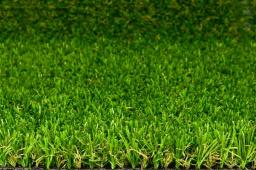We would be pleased if you find an error in the word problem, spelling mistakes, or inaccuracies and send it to us. Thank you!#### You need to know the following knowledge to solve this word math problem:

We encourage you to watch this tutorial video on this math problem:

## Next similar math problems:

• FlowerbedIn the park there is a large circular flowerbed with a diameter of 12 m. Jakub circulated him ten times and the smaller Vojtoseven times. How many meters each went by and how many meters did Jakub run more than Vojta?
• Round flowerbed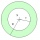Around a round flowerbed with a diameter of 6 meters and I will make a sidewalk up to 0.5 meters wide. How much gravel is needed if the layer is to be 5 cm high?
• HectareThe rectangular plot has a width of 2500 cm and is 0.065 km long. How many CZK will we pay for covering the land with a lawn at the price of CZK 3,500 per hectare?
• A rectangularA rectangular garden 40 m long and 30 m wide is to be fenced with fence posts at each corner. All the other posts will be 5 meters a part. How many posts will be needed to fence in the garden.
• Natural fertilizerThe rectangular garden measuring 120m and 60m was fertilized with 16kg of natural fertilizer. Natural fertilizer contains 45% organic matter. How much organic matter falls on 1 m2 of garden?
• Pool tilesThe pool is 25m long, 10m wide and 160cm deep. How many m2 of tiles will be needed on the walls and the pool? How many pieces of tile are needed when 1 tile has a square shape with a 20cm side? How much does it cost when 1m2 of tiles costs 258 Kc?
• Grass seeds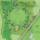How many kg of grass seed is needed to be sowing a circular field with a diameter of 120 meters when the 1 m2 should be used 0.8 grams seeds?
• Circular flowerbed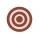Circular flowerbed with diameter 8 m we split by concentric circle to circle and annulus with the same area. Determine the radius of the circle.
• The carpet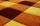How many meters of carpet 90 cm wide need to cover floor room which has a rectangular shape with a lengths 4.8 m and 2.4 m if the number of pieces on the carpet is needed to be lowest?
• Folding tableThe folding kitchen table has a rectangular shape with an area of 168dm2 (side and is 14 dm long). If necessary, it can be enlarged by sliding two semi-circular plates (at sides b). How much percent will the table area increase? The result round to one-hu
• Sand pathHow many m3 of sand is needed to fill the 1.5m wide path around a rectangular flowerbed of 8m and 14m if the sand layer is 6cm high?
• Circular lawnAround a circular lawn area is 2 m wide sidewalk. The outer edge of the sidewalk is curb whose width is 2 m. Curbstone and the inner side of the sidewalk together form a concentric circles. Calculate the area of the circular lawn and the result round to 1
• TrevorTrevor wishes to tile the floor of his bathroom. The bathroom is rectangular and is 4.2m long and 3.3m wide. The tiles are 30cm by 30cm. Calculate the cost to tile the floor if each tile costs 72 cents.
• HallRectangular hall will have pave by square tiles with a side length 15 cm. The hall has length 18 meters and width 3 m. How many tiles need to buy if 2 percent of the amount is disrupted during the work?
• Office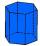Office building was built in the shape of a regular hexagon inscribed in a circle with a radius of 12 m. The height of the walls is 7m. How much CZK cost plastering the walls of the building, if per 1 m square cost CZK 400?
• SidewalkThe city park is a circular bed of flowers with a diameter of 8 meters, around it the whole length is 1 meter wide sidewalk . What is the sidewalk area?
• Square gardensThe gardening colony with dimensions of 180 m and 300 m is to be completely divided into equally large square areas with the largest possible area. Calculate how many such square areas can be obtained and determine the side length of the square.